# Maharashtra SSC Board Solved Maths 2019 Question Paper 1

Previous year question papers play an important role in board exam preparation. Especially a subject like Maths, which demands a lot of practice has to be solved by students. Practising the Maharashtra Board Class 10 Previous Year Question Paper helps students to understand the types of questions asked in the exam and their difficulty level. So, to help students prepare well for the SSC Board exam, we are providing the MSBSHSE Class 10 Solved Maths 2019 Previous Year Question Paper.

## Maharashtra SSC Board Solved Maths 2019 Question Paper 1 – Download Solutions

Students can download the Maharashtra SSC Board Solved Maths 2019 Part 1 Paper from the table below. We have also provided the Part 1 Maths Question Paper 2019.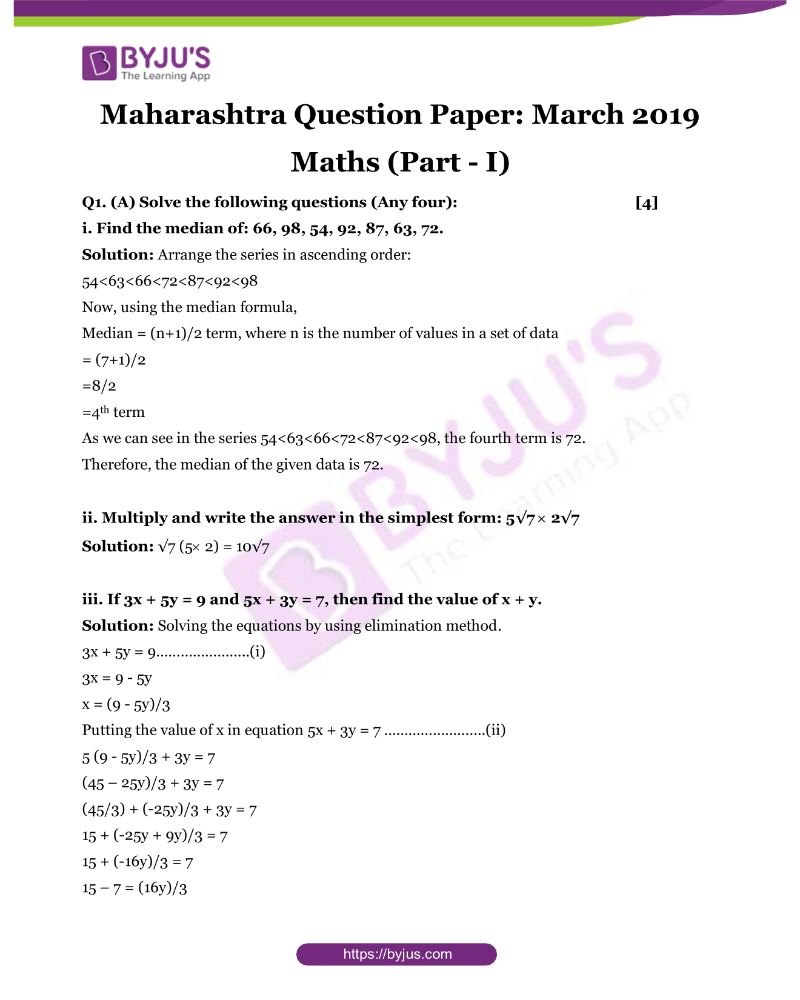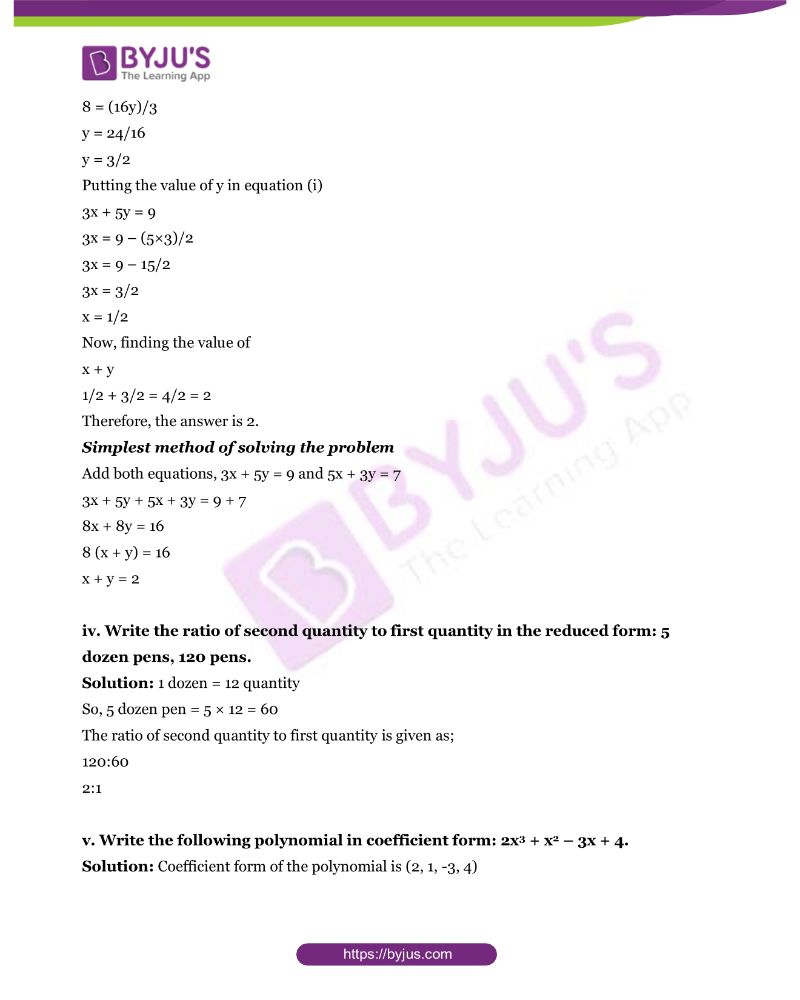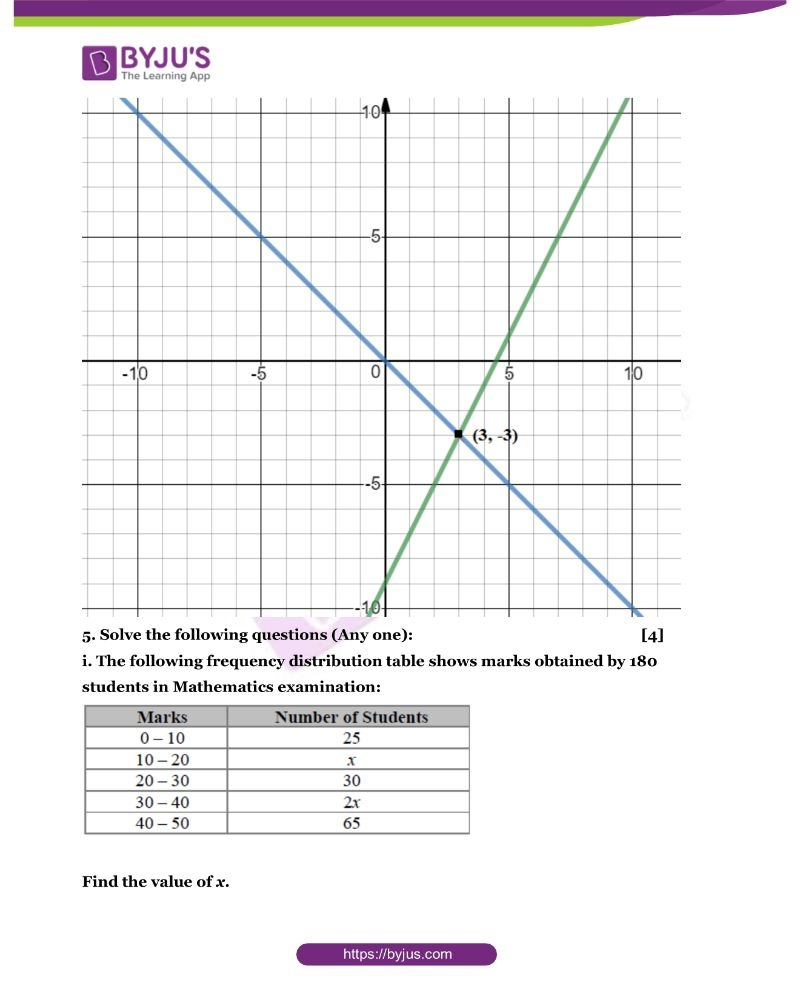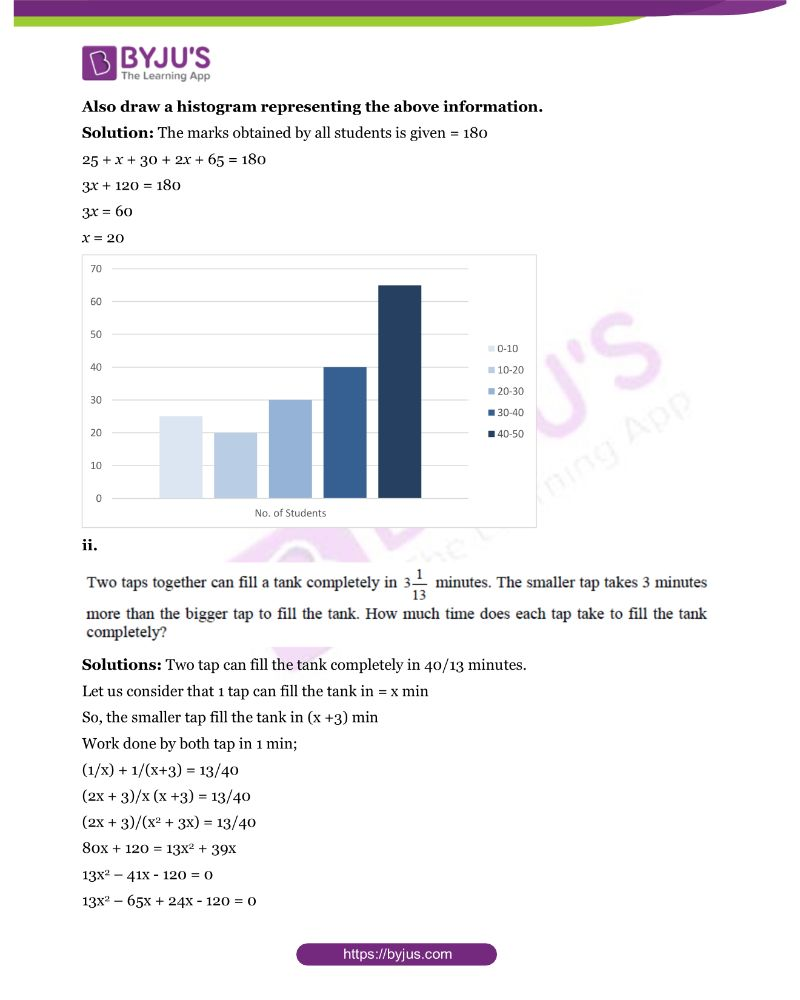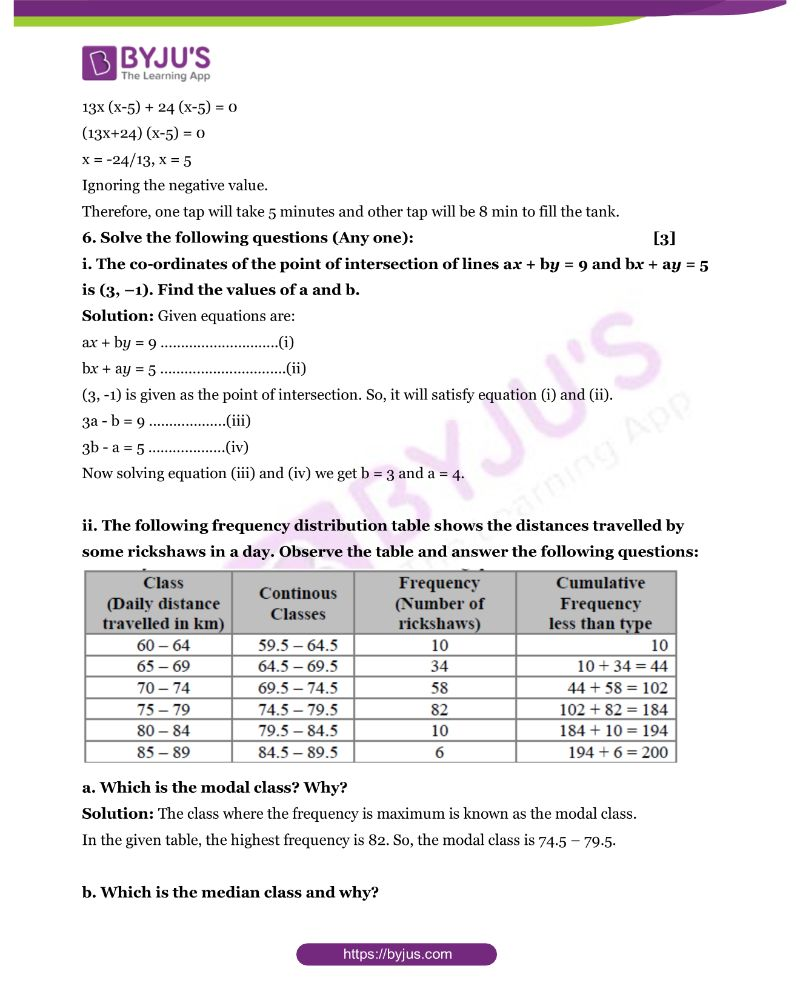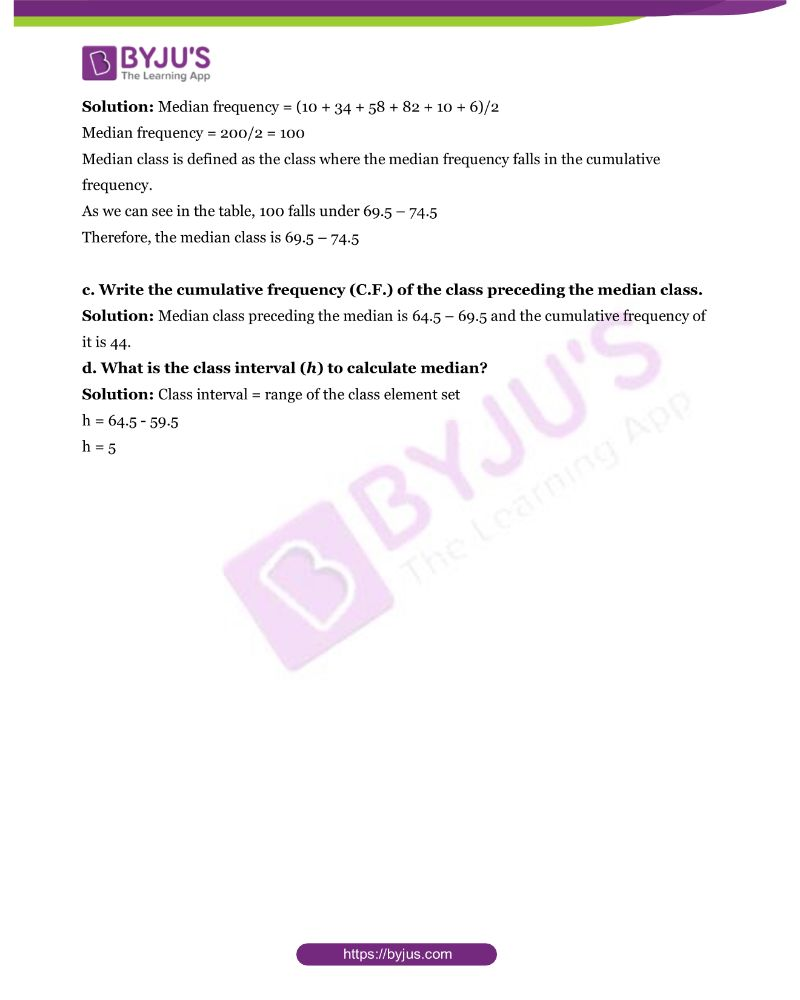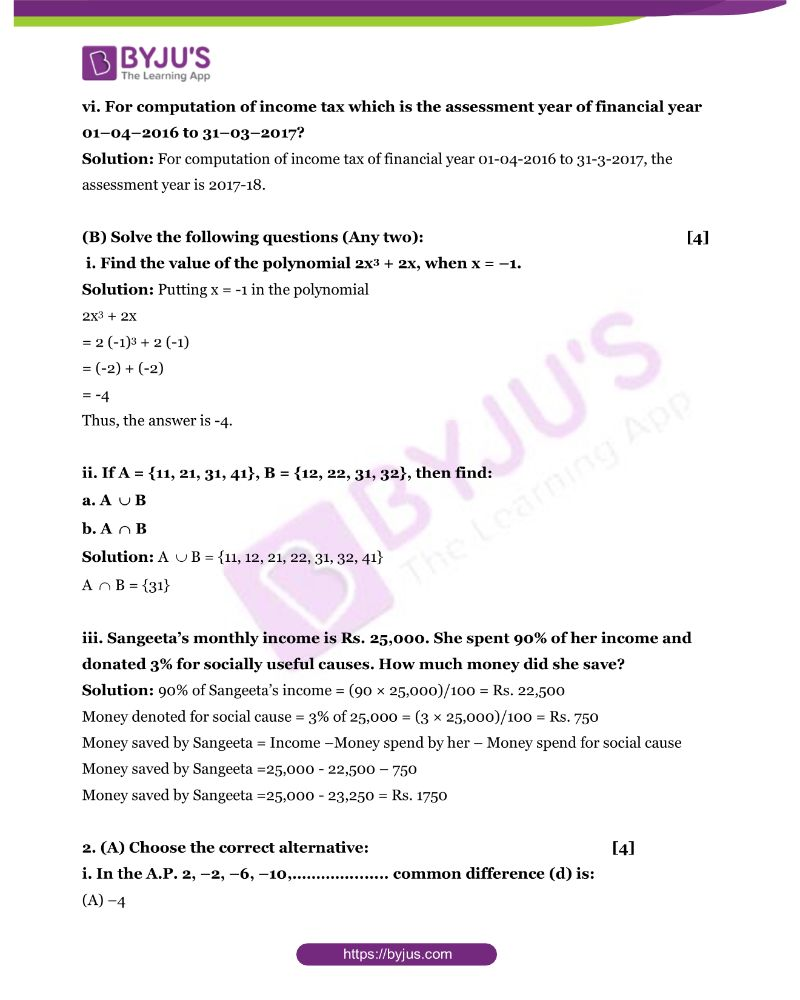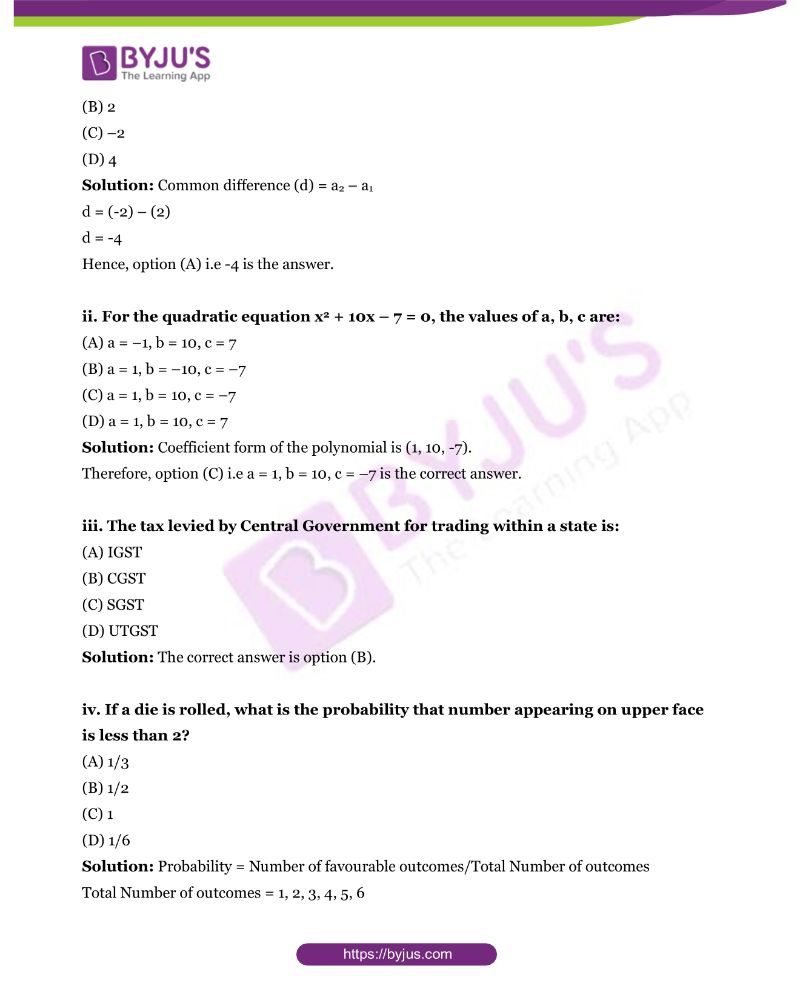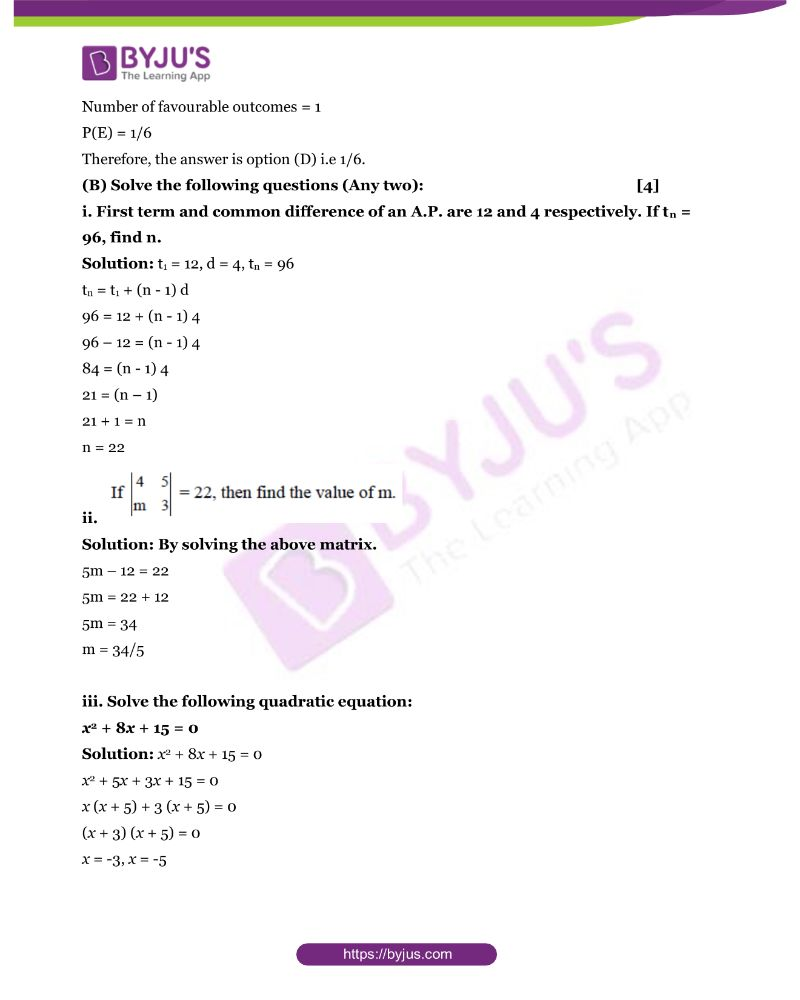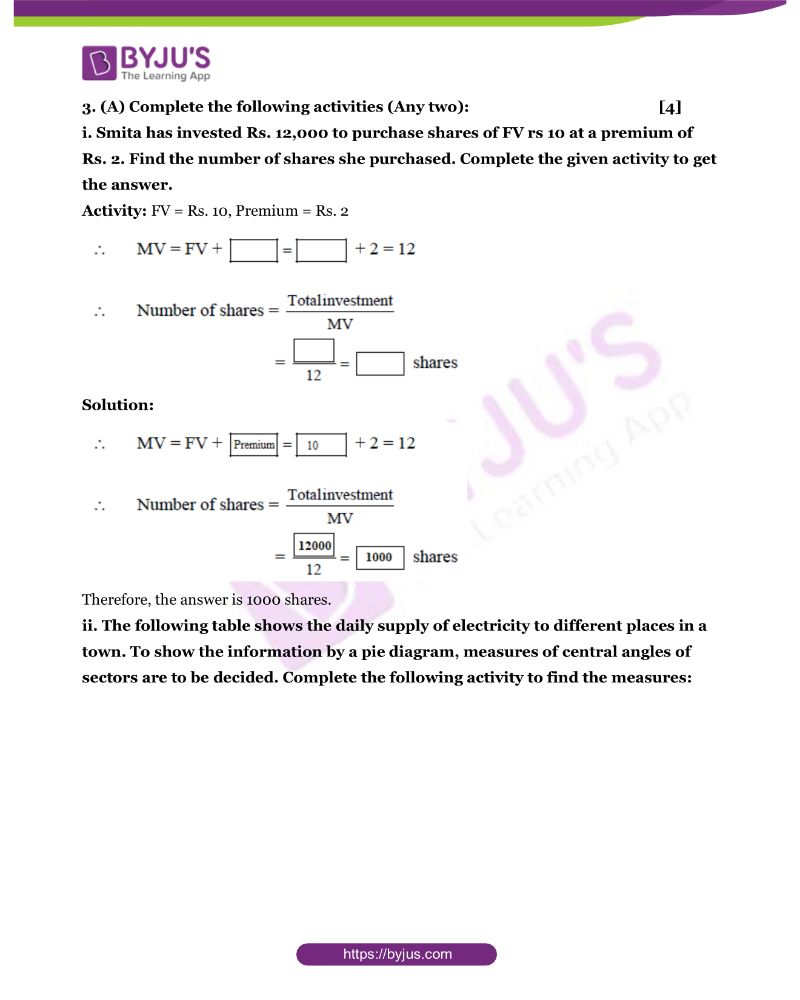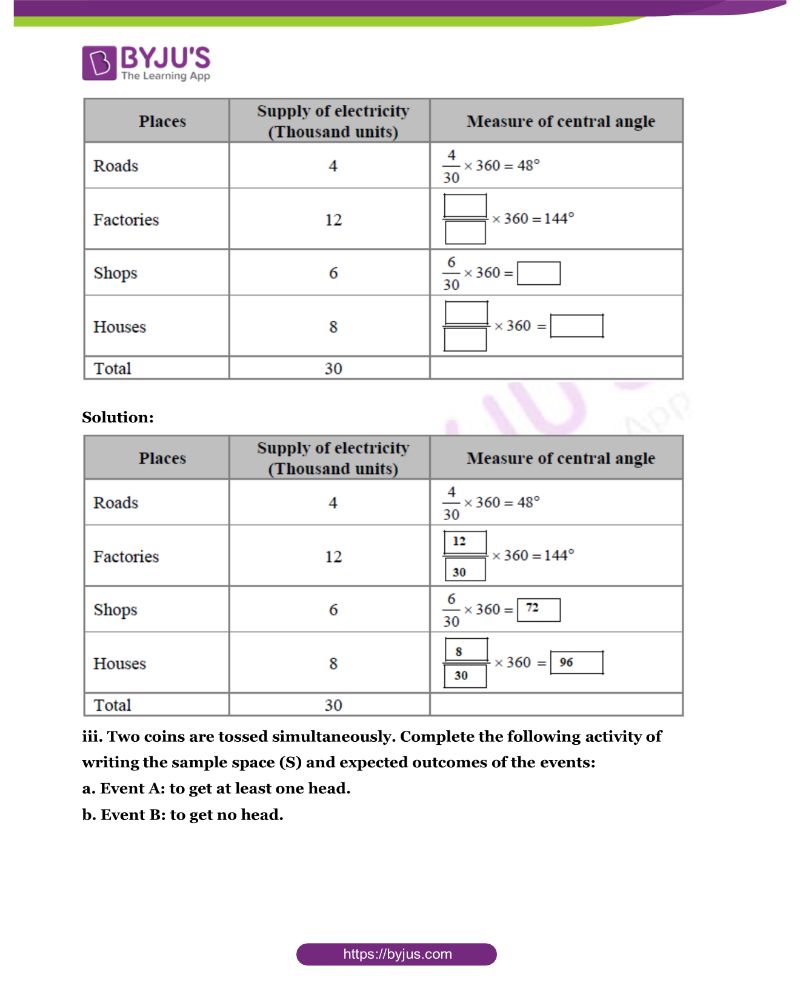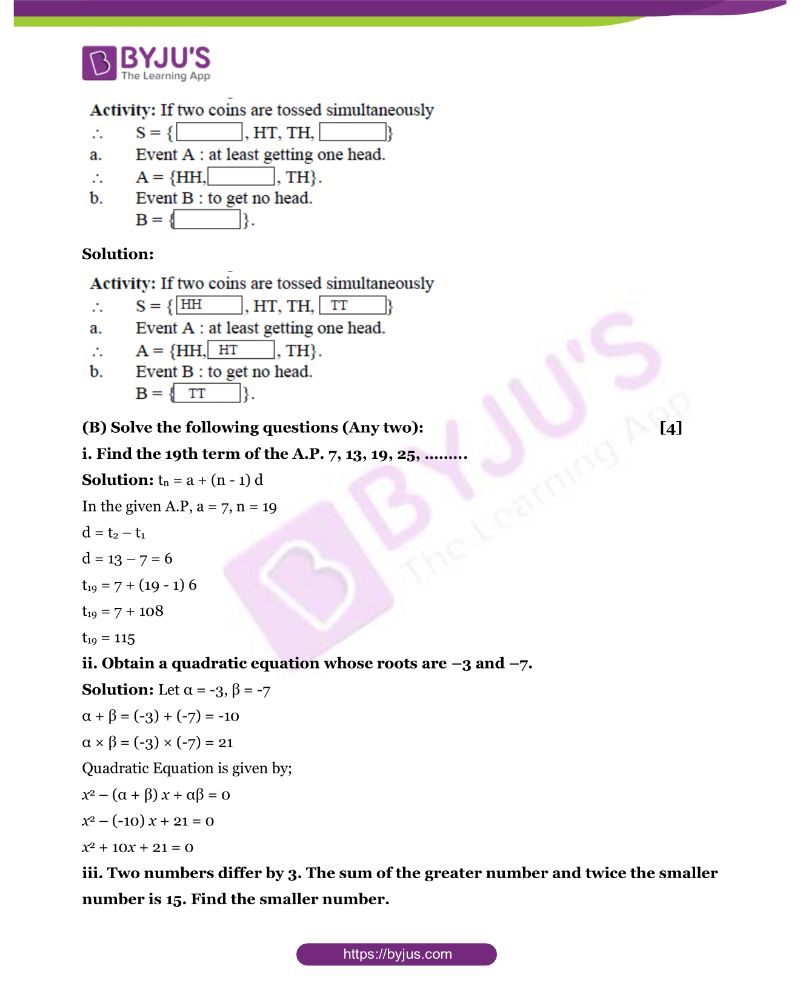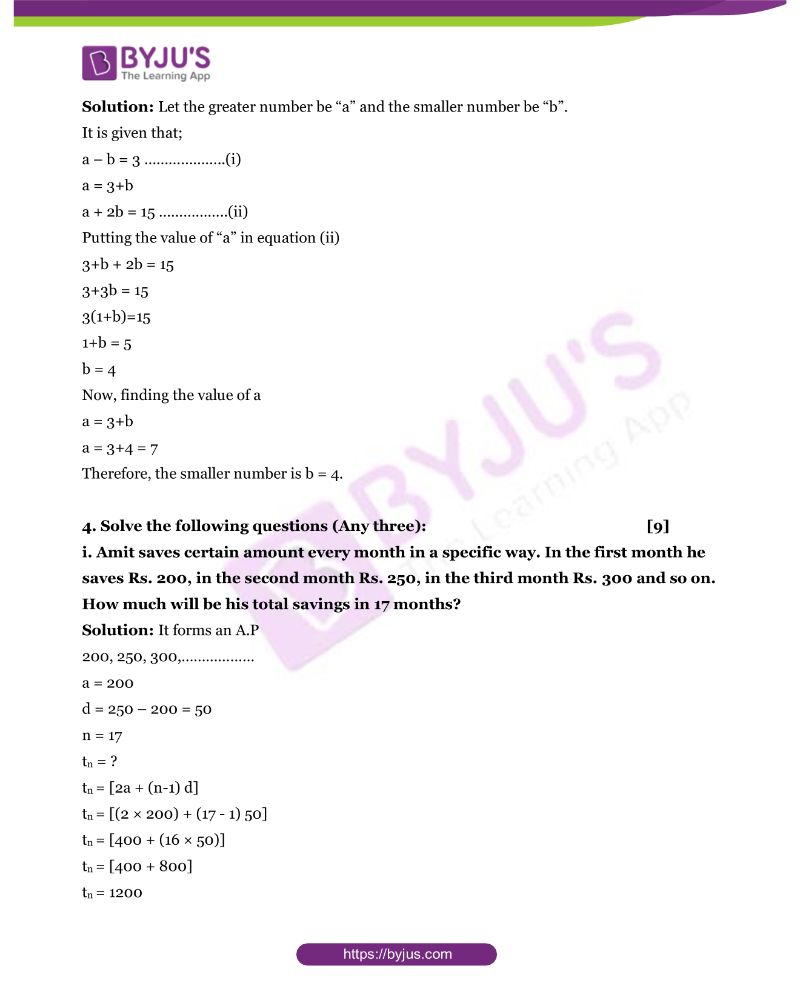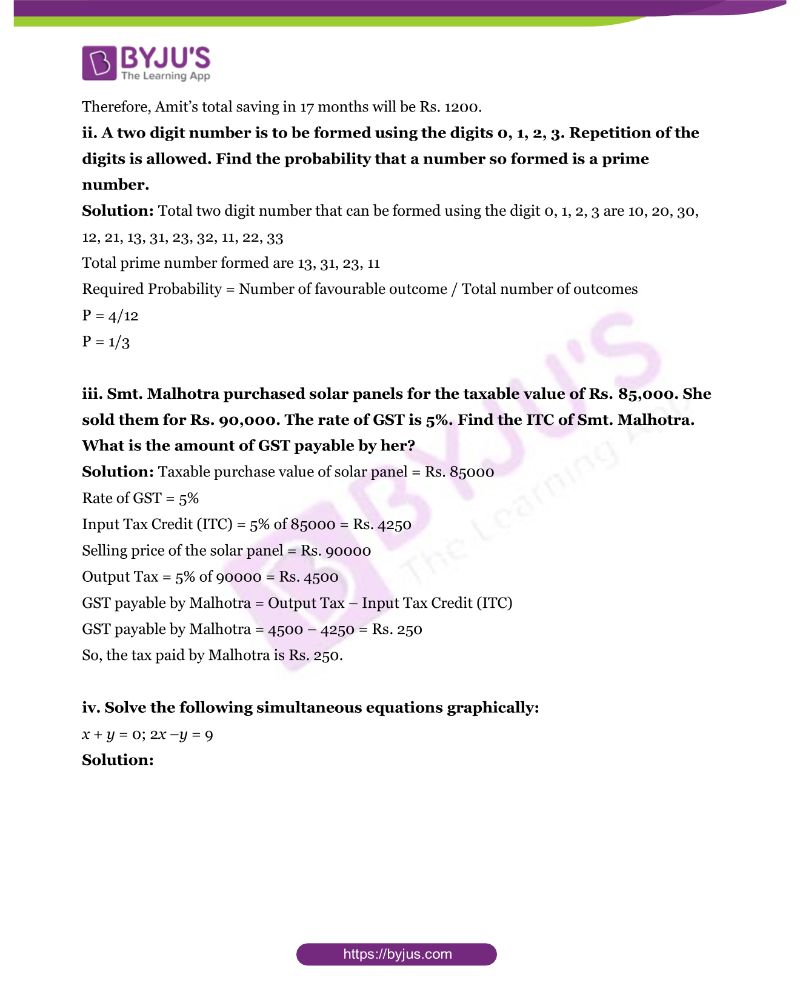## MSBSHSE Class 10 Maths Part 1 Questions with Solutions

Q1. (A) Solve the following questions (Any four): 

i. Find the median of: 66, 98, 54, 92, 87, 63, 72.

Solution: Arrange the series in ascending order:

54<63<66<72<87<92<98

Now, using the median formula,

Median = (n+1)/2 term, where n is the number of values in a set of data

= (7+1)/2

=8/2

=4th term

As we can see in the series 54<63<66<72<87<92<98, the fourth term is 72.

Therefore, the median of the given data is 72.

ii. Multiply and write the answer in the simplest form: 5√7 × 2√7

Solution: √7 (5× 2) = 10√7

iii. If 3x + 5y = 9 and 5x + 3y = 7, then find the value of x + y.

Solution: Solving the equations by using elimination method.

3x + 5y = 9…………………..(i)

3x = 9 – 5y

x = (9 – 5y)/3

Putting the value of x in equation 5x + 3y = 7 …………………….(ii)

5 (9 – 5y)/3 + 3y = 7

(45 – 25y)/3 + 3y = 7

(45/3) + (-25y)/3 + 3y = 7

15 + (-25y + 9y)/3 = 7

15 + (-16y)/3 = 7

15 – 7 = (16y)/3

8 = (16y)/3

y = 24/16

y = 3/2

Putting the value of y in equation (i)

3x + 5y = 9

3x = 9 – (5×3)/2

3x = 9 – 15/2

3x = 3/2

x = 1/2

Now, finding the value of

x + y

1/2 + 3/2 = 4/2 = 2

Simplest method of solving the problem

Add both equations, 3x + 5y = 9 and 5x + 3y = 7

3x + 5y + 5x + 3y = 9 + 7

8x + 8y = 16

8 (x + y) = 16

x + y = 2

iv. Write the ratio of second quantity to first quantity in the reduced form: 5 dozen pens, 120 pens.

Solution: 1 dozen = 12 quantity

So, 5 dozen pen = 5 × 12 = 60

The ratio of second quantity to first quantity is given as;

120:60

2:1

v. Write the following polynomial in coefficient form: 2x3 + x2 – 3x + 4.

Solution: Coefficient form of the polynomial is (2, 1, -3, 4)

vi. For computation of income tax which is the assessment year of financial year 01–04–2016 to 31–03–2017?

Solution: For computation of income tax of financial year 01-04-2016 to 31-3-2017, the assessment year is 2017-18.

(B) Solve the following questions (Any two): 

i. Find the value of the polynomial 2x3 + 2x, when x = –1.

Solution: Putting x = -1 in the polynomial

2x3 + 2x

= 2 (-1)3 + 2 (-1)

= (-2) + (-2)

= -4

ii. If A = {11, 21, 31, 41}, B = {12, 22, 31, 32}, then find:

a. A ∪ B

b. A ∩ B

Solution: A ∪ B = {11, 12, 21, 22, 31, 32, 41}

A ∩ B = {31}

iii. Sangeeta’s monthly income is Rs. 25,000. She spent 90% of her income and donated 3% for socially useful causes. How much money did she save?

Solution: 90% of Sangeeta’s income = (90 × 25,000)/100 = Rs. 22,500

Money denoted for social cause = 3% of 25,000 = (3 × 25,000)/100 = Rs. 750

Money saved by Sangeeta = Income –Money spend by her – Money spend for social cause

Money saved by Sangeeta =25,000 – 22,500 – 750

Money saved by Sangeeta =25,000 – 23,250 = Rs. 1750

2. (A) Choose the correct alternative: 

i. In the A.P. 2, –2, –6, –10,……………….. common difference (d) is:

(A) –4

(B) 2

(C) –2

(D) 4

Solution: Common difference (d) = a2 – a1

d = (-2) – (2)

d = -4

Hence, option (A) i.e -4 is the answer.

ii. For the quadratic equation x2 + 10x – 7 = 0, the values of a, b, c are:

(A) a = –1, b = 10, c = 7

(B) a = 1, b = –10, c = –7

(C) a = 1, b = 10, c = –7

(D) a = 1, b = 10, c = 7

Solution: Coefficient form of the polynomial is (1, 10, -7).

Therefore, option (C) i.e a = 1, b = 10, c = –7 is the correct answer.

iii. The tax levied by Central Government for trading within a state is:

(A) IGST

(B) CGST

(C) SGST

(D) UTGST

Solution: The correct answer is option (B).

iv. If a die is rolled, what is the probability that number appearing on upper face is less than 2?

(A) 1/3

(B) 1/2

(C) 1

(D) 1/6

Solution: Probability = Number of favourable outcomes/Total Number of outcomes

Total Number of outcomes = 1, 2, 3, 4, 5, 6

Number of favourable outcomes = 1

P(E) = 1/6

Therefore, the answer is option (D) i.e 1/6.

(B) Solve the following questions (Any two): 

i. First term and common difference of an A.P. are 12 and 4 respectively. If tn = 96, find n.

Solution: t1 = 12, d = 4, tn = 96

tn = t1 + (n – 1) d

96 = 12 + (n – 1) 4

96 – 12 = (n – 1) 4

84 = (n – 1) 4

21 = (n – 1)

21 + 1 = n

n = 22

ii.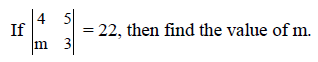Solution: By solving the above matrix.

5m – 12 = 22

5m = 22 + 12

5m = 34

m = 34/5

iii. Solve the following quadratic equation:

x2 + 8x + 15 = 0

Solution: x2 + 8x + 15 = 0

x2 + 5x + 3x + 15 = 0

x (x + 5) + 3 (x + 5) = 0

(x + 3) (x + 5) = 0

x = -3, x = -5

3. (A) Complete the following activities (Any two): 

i. Smita has invested Rs. 12,000 to purchase shares of FV rs 10 at a premium of Rs. 2. Find the number of shares she purchased. Complete the given activity to get the answer.

Activity: FV = Rs. 10, Premium = Rs. 2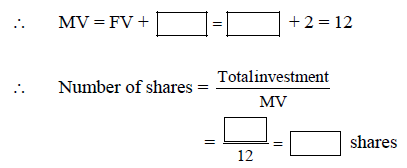Solution: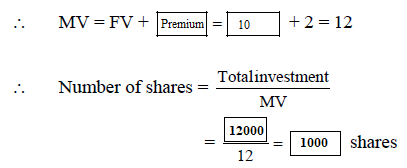Therefore, the answer is 1000 shares.

ii. The following table shows the daily supply of electricity to different places in a town. To show the information by a pie diagram, measures of central angles of sectors are to be decided. Complete the following activity to find the measures: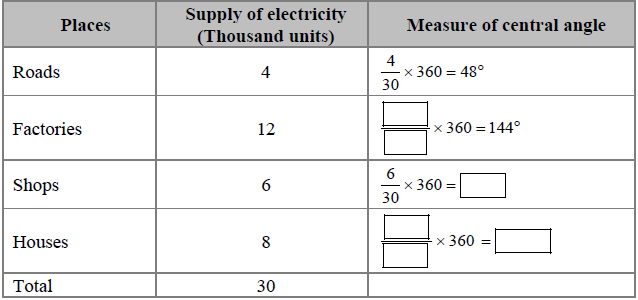Solution: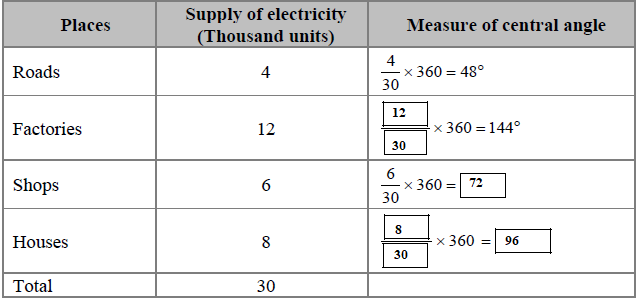iii. Two coins are tossed simultaneously. Complete the following activity of writing the sample space (S) and expected outcomes of the events:

a. Event A: to get at least one head.

b. Event B: to get no head.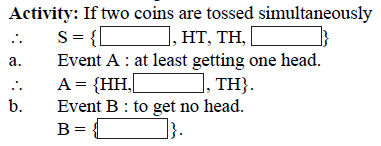Solution: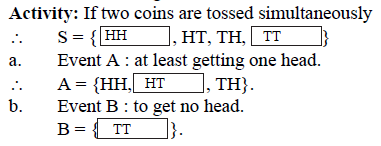(B) Solve the following questions (Any two): 

i. Find the 19th term of the A.P. 7, 13, 19, 25, ………

Solution: tn = a + (n – 1) d

In the given A.P, a = 7, n = 19

d = t2 – t1

d = 13 – 7 = 6

t19 = 7 + (19 – 1) 6

t19 = 7 + 108

t19 = 115

ii. Obtain a quadratic equation whose roots are –3 and –7.

Solution: Let α = -3, β = -7

α + β = (-3) + (-7) = -10

α × β = (-3) × (-7) = 21

x2 – (α + β) x + αβ = 0

x2 – (-10) x + 21 = 0

x2 + 10x + 21 = 0

iii. Two numbers differ by 3. The sum of the greater number and twice the smaller number is 15. Find the smaller number.

Solution: Let the greater number be “a” and the smaller number be “b”.

It is given that;

a – b = 3 ………………..(i)

a = 3+b

a + 2b = 15 ……………..(ii)

Putting the value of “a” in equation (ii)

3+b + 2b = 15

3+3b = 15

3(1+b)=15

1+b = 5

b = 4

Now, finding the value of a

a = 3+b

a = 3+4 = 7

Therefore, the smaller number is b = 4.

4. Solve the following questions (Any three): 

i. Amit saves certain amount every month in a specific way. In the first month he saves Rs. 200, in the second month Rs. 250, in the third month Rs. 300 and so on. How much will be his total savings in 17 months?

Solution: It forms an A.P

200, 250, 300,………………

a = 200

d = 250 – 200 = 50

n = 17

tn = ?

tn = [2a + (n-1) d]

tn = [(2 × 200) + (17 – 1) 50]

tn = [400 + (16 × 50)]

tn = [400 + 800]

tn = 1200

Therefore, Amit’s total saving in 17 months will be Rs. 1200.

ii. A two digit number is to be formed using the digits 0, 1, 2, 3. Repetition of the digits is allowed. Find the probability that a number so formed is a prime number.

Solution: Total two digit number that can be formed using the digit 0, 1, 2, 3 are 10, 20, 30, 12, 21, 13, 31, 23, 32, 11, 22, 33

Total prime number formed are 13, 31, 23, 11

Required Probability = Number of favourable outcome / Total number of outcomes

P = 4/12

P = 1/3

iii. Smt. Malhotra purchased solar panels for the taxable value of Rs. 85,000. She sold them for Rs. 90,000. The rate of GST is 5%. Find the ITC of Smt. Malhotra. What is the amount of GST payable by her?

Solution: Taxable purchase value of solar panel = Rs. 85000

Rate of GST = 5%

Input Tax Credit (ITC) = 5% of 85000 = Rs. 4250

Selling price of the solar panel = Rs. 90000

Output Tax = 5% of 90000 = Rs. 4500

GST payable by Malhotra = Output Tax – Input Tax Credit (ITC)

GST payable by Malhotra = 4500 – 4250 = Rs. 250

So, the tax paid by Malhotra is Rs. 250.

iv. Solve the following simultaneous equations graphically:

x + y = 0; 2x y = 9

Solution: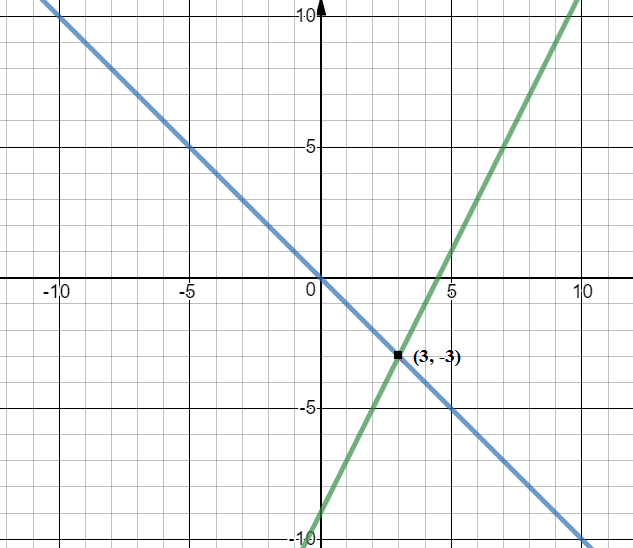5. Solve the following questions (Any one): 

i. The following frequency distribution table shows marks obtained by 180 students in Mathematics examination: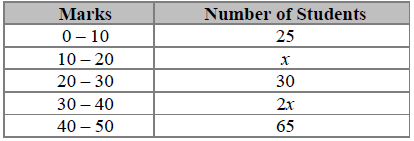Find the value of x.

Also draw a histogram representing the above information.

Solution: The marks obtained by all students is given = 180

25 + x + 30 + 2x + 65 = 180

3x + 120 = 180

3x = 60

x = 20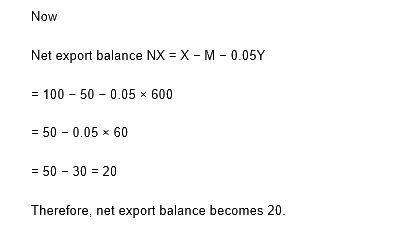ii.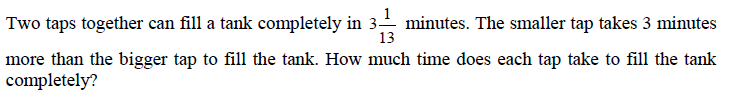Solutions: Two tap can fill the tank completely in 40/13 minutes.

Let us consider that 1 tap can fill the tank in = x min

So, the smaller tap fill the tank in (x +3) min

Work done by both tap in 1 min;

(1/x) + 1/(x+3) = 13/40

(2x + 3)/x (x +3) = 13/40

(2x + 3)/(x2 + 3x) = 13/40

80x + 120 = 13x2 + 39x

13x2 – 41x – 120 = 0

13x2 – 65x + 24x – 120 = 0

13x (x-5) + 24 (x-5) = 0

(13x+24) (x-5) = 0

x = -24/13, x = 5

Ignoring the negative value.

Therefore, one tap will take 5 minutes and other tap will be 8 min to fill the tank.

6. Solve the following questions (Any one): 

i. The co-ordinates of the point of intersection of lines ax + by = 9 and bx + ay = 5 is (3, –1). Find the values of a and b.

Solution: Given equations are:

ax + by = 9 ………………………..(i)

bx + ay = 5 ………………………….(ii)

(3, -1) is given as the point of intersection. So, it will satisfy equation (i) and (ii).

3a – b = 9 ……………….(iii)

3b – a = 5 ……………….(iv)

Now solving equation (iii) and (iv) we get b = 3 and a = 4.

ii. The following frequency distribution table shows the distances travelled by some rickshaws in a day. Observe the table and answer the following questions: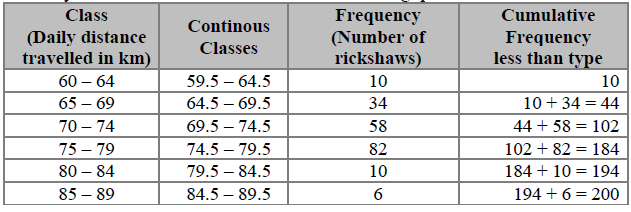a. Which is the modal class? Why?

Solution: The class where the frequency is maximum is known as the modal class.

In the given table, the highest frequency is 82. So, the modal class is 74.5 – 79.5.

b. Which is the median class and why?

Solution: Median frequency = (10 + 34 + 58 + 82 + 10 + 6)/2

Median frequency = 200/2 = 100

Median class is defined as the class where the median frequency falls in the cumulative frequency.

As we can see in the table, 100 falls under 69.5 – 74.5

Therefore, the median class is 69.5 – 74.5

c. Write the cumulative frequency (C.F.) of the class preceding the median class.

Solution: Median class preceding the median is 64.5 – 69.5 and the cumulative frequency of it is 44.

d. What is the class interval (h) to calculate median?

Solution: Class interval = range of the class element set

h = 64.5 – 59.5

h = 5

Register with BYJU’S and get latest updates on States Board, CBSE, ICSE and competitive exams.